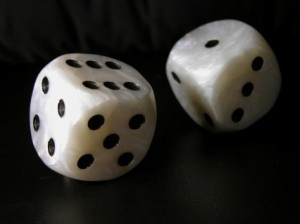## Random Variables: Why bother?

Categories: Building Blocks
Tags: No Tags
Published on: July 14, 2012

Random variables and their probability distributions are the basis of inferential statistics.

1. In the exploration of most concepts in life and in science, they can be characterised as one of two types of processes: A. Deterministic Processes. B. Random Processes. Random processes produce random variable. The random variable is also referred to as a stochastic variable. Deterministic process produces deterministic variables.

1.1. A random process is one in which the outcomes are entirely random or unpredictable. The outcome is unknown and not fixed, but can have a set of values depending on the process producing it. A coin toss can have one of two values; the child’s sex at birth also has two possibilities male or female , a roll of a dice any one of six possibilities, the disposition of the patient at discharge can have X values with probability of p(X), expressed as relative frequencies stabilising in the long run.2. A deterministic variable simply means that it is not random .A deterministic process behaves as a mathematical function and thus repeatedly produces a predictable outcome depending on the particular inputs.

2.1 However, in deterministic process even though they produce predictable outcomes [i.e. Deterministic variables] , there always a small degree of random behaviour: for example due to error in the measuring instrument or otherwise called measurement error. Historically, it the analysis of this error or the theory of errors, that evolved into the field of statistics.

3. In the case of purely random processes and that small part of deterministic processes made up of random errors, the variables cannot be characterized individually, but will follow some probability distribution.

3.1. The value of the random variable can never be known in advance. However we can know the set of possible values. Eg in the role of a dice, the possibility is 1 to six as discussed in 1.1. This information is given to us from the probability distribution of this random variable, made up of the long run relative frequencies of each possible value.

3.1. Such a probability distribution will describe the relative frequencies of occurrence of different-sized errors in the case of the random compartment of deterministic processes.

4. QUANTITATIVE RANDOM VARIABLES

A random quantitative variable results when numerical values are assigned to results of measurement or counting. It is called a discrete random variable if the assignment is based on counting. It is called a continuous random variable if the numerical assignment is based on measurement.

The numerical continuous random variable can be expressed as fractions and decimals. The numerical discrete random variable can only be expressed as whole numbers.

4.1QUALITATIVE RANDOM VARIABLES

Qualitative variables (nominal, ordinal, and ranked) are attribute or categorical with no intrinsic numerical value. The nominal has no ordering for example male or female. The ordinal has ordering for example first class, second class, third class. The ranked has observations arrayed in ascending or descending orders of magnitude for example 1st, 2nd, 3rd.

4.2. RANDOM VARIABLES: MATHEMATICAL TRANSFORMATIONS

Quantitative variables can be transformed into qualitative ones. Qualitative variables can be transformed into quantitative ones but this is less desirable. The continuous variable can be transformed into the discrete variable. Transformation of the discrete into the continuous may be misleading.

5. RANDOM VARIABLES: PROPERTIES

A random variable has 6 properties. The expectation or average of a random variable is a central value around which it hovers most of the time. The variations of the random variable around the average are measured by its variance. Covariance measures the co-variability of the two random variables. Correlation measures the linear relation between two random variables. Skew ness measures the bias of the distribution of the random variable from the center. Kurtosis measures the peaked ness of the random variable is at the point of its expectation.

5.1

Welcome , today is Thursday, January 21, 2021